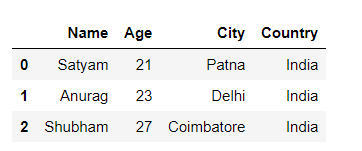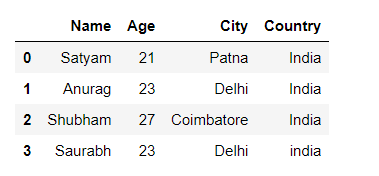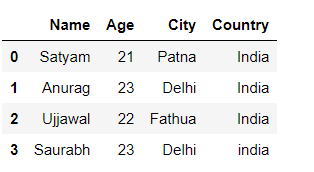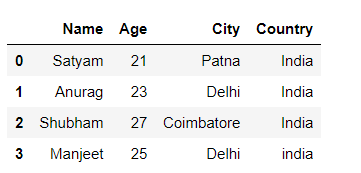Related Articles

# How to append a list as a row to a Pandas DataFrame in Python?

• Last Updated : 05 Oct, 2021

Prerequisite: Pandas DataFrame

In this article, We are going to see how to append a list as a row to a pandas dataframe in Python. It can be done in three ways:

## Append list using loc[] methods

Pandas DataFrame.loc attribute access a group of rows and columns by label(s) or a boolean array in the given DataFrame.

Let’s append the list with step-wise:

Step 1: Create a simple dataframe using the list.

## Python3

 `import` `pandas as pd` `# List``Person ``=` `[ [``'Satyam'``, ``21``, ``'Patna'` `, ``'India'` `],``            ``[``'Anurag'``, ``23``, ``'Delhi'` `, ``'India'` `],``            ``[``'Shubham'``, ``27``, ``'Coimbatore'` `, ``'India'` `]]` `#Create a DataFrame object``df ``=` `pd.DataFrame(Person,``                  ``columns ``=` `[``'Name'` `, ``'Age'``, ``'City'` `, ``'Country'``])` `# display``display(df)`

Output:Step 2: Using loc to append the new list to a data frame.

## Python3

 `# New list for append into df``list` `=` `[``"Saurabh"``, ``23``, ``"Delhi"``, ``"india"``]` `# using loc methods``df.loc[``len``(df)] ``=` `list` `# display``display(df)`

Output:## Append list using iloc[] methods

Pandas DataFrame.iloc method access integer-location based indexing for selection by position.

Example:

## Python3

 `# import module``import` `pandas as pd` `# List``Person ``=` `[ [``'Satyam'``, ``21``, ``'Patna'` `, ``'India'` `],``            ``[``'Anurag'``, ``23``, ``'Delhi'` `, ``'India'` `],``            ``[``'Shubham'``, ``27``, ``'Coimbatore'` `, ``'India'` `],``            ``[``"Saurabh"``, ``23``, ``"Delhi"``, ``"india"``]]` `#Create a DataFrame object``df ``=` `pd.DataFrame(Person,``                  ``columns ``=` `[``'Name'` `, ``'Age'``, ``'City'` `, ``'Country'``])` `# new list to append into df``list` `=` `[``'Ujjawal'``, ``22``, ``'Fathua'``, ``'India'``]` `# using iloc``df.iloc[``2``] ``=` `list` `# display``display(df)`

Output:Note – It is used for location-based indexing so it works for only the existing index and replaces the row element.

## Append list using append() methods

Pandas dataframe.append() function is used to append rows of other dataframe to the end of the given dataframe, returning a new dataframe object.

Example:

## Python3

 `# import module``import` `pandas as pd` `# List``Person ``=` `[ [``'Satyam'``, ``21``, ``'Patna'` `, ``'India'` `],``            ``[``'Anurag'``, ``23``, ``'Delhi'` `, ``'India'` `],``            ``[``'Shubham'``, ``27``, ``'Coimbatore'` `, ``'India'` `]]` `#Create a DataFrame object``df ``=` `pd.DataFrame(Person,``                  ``columns ``=` `[``'Name'` `, ``'Age'``, ``'City'` `, ``'Country'``])` `# new list to append into df``list` `=` `[[``"Manjeet"``, ``25``, ``"Delhi"``, ``"india"``]]` `# using append``df ``=` `df.append(pd.DataFrame( ``list``,``               ``columns``=``[ ``'Name'``, ``'Age'``, ``'City'``, ``'Country'``]),``               ``ignore_index ``=` `True``)` `# display df``display(df)`

Output:Attention geek! Strengthen your foundations with the Python Programming Foundation Course and learn the basics.

To begin with, your interview preparations Enhance your Data Structures concepts with the Python DS Course. And to begin with your Machine Learning Journey, join the Machine Learning – Basic Level Course

My Personal Notes arrow_drop_up